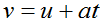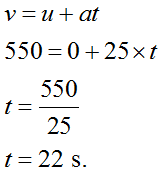# If an object is accelerating at a rate of 25 m/s2, how long (in seconds) will it take to reach a speed of 550 m/s? (Assume an initial velocity of zero.)Group of answer choices

Question
66 views
If an object is accelerating at a rate of 25 m/s2, how long (in seconds) will it take to reach a speed of 550 m/s? (Assume an initial velocity of zero.)

check_circle

Step 1

Given:

Accelerating rate (a) = 25 m/s2.

Final velocity (v) = 550 m/s.

Initial velocity (u) = 0 m/s.

Step 2

The first equation of kinematic is,where,

v = final velocity.

u = initial velocity.

a = acceleration.

t = time.

Step 3

Time taken by an object to reach at 550 m/s,...

### Want to see the full answer?

See Solution

#### Want to see this answer and more?

Solutions are written by subject experts who are available 24/7. Questions are typically answered within 1 hour.*

See Solution
*Response times may vary by subject and question.
Tagged in